# Answer of quiz | Online Quiz on “Data Structure using C” | Free Mar Points

1) C is a: *

General Purpose Programming Language

2) Which of the following are valid data types in C? *
int, float, double, char
3) What is the starting point for a C program? *
The main() function
4) The preprocessor acts: *
Before compilation
5) Which one of the following is not a reserved keyword in C? *
main
6) What will be printed after execution of following code? *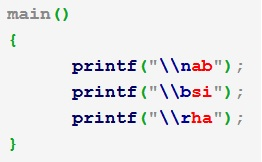hai
7) What will be the output of the following program? *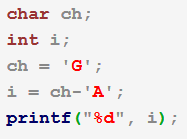6
8) Find out the output of the following program: *
Syntax eroor
9) What is the difference between declaration and definition of a variable ? *
There is no difference between them.
10) What will happen after compiling and running the following code? *
11) What will be the output of the following code? *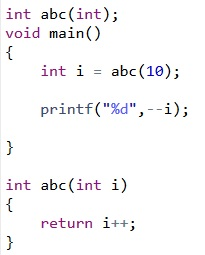9
12) Determine the output? *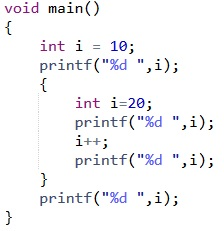10 20 21 10
13) Find out the output of the following program *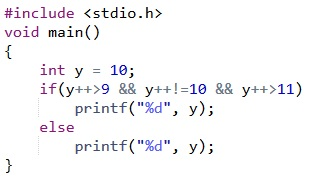7) What will be the output of the following C program? *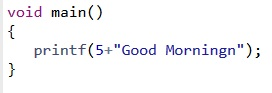Morningn
18) What is the return type of a method that does not return any value? *
void
19) Which of the following operator take only integer operand ? *
%
20) What will be the output of the following C program? *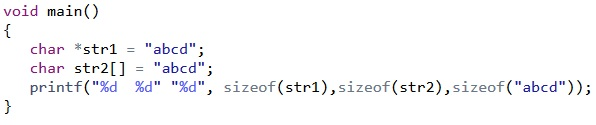8 5 5